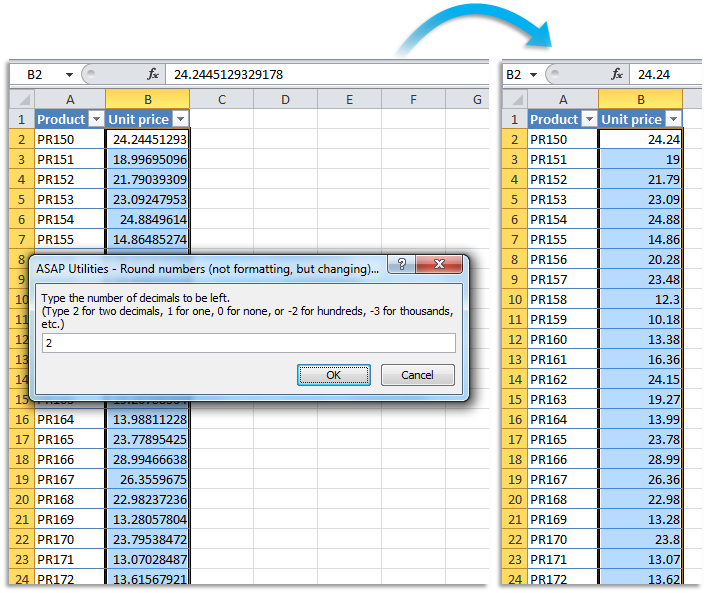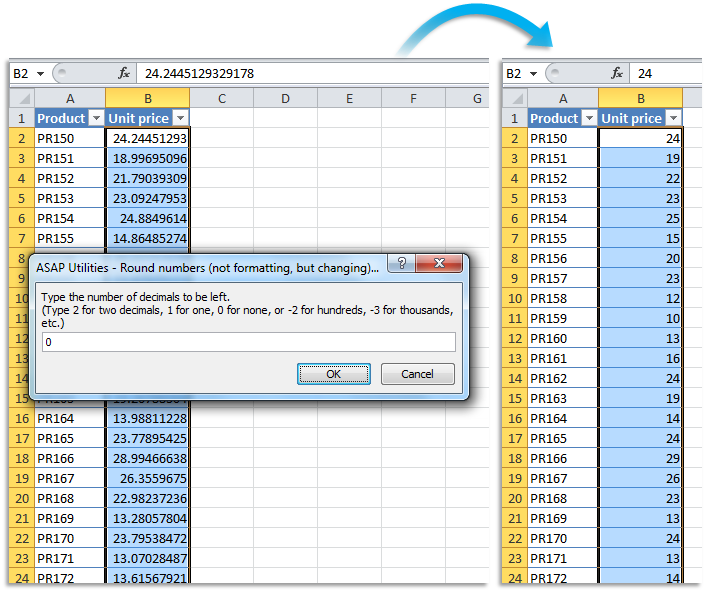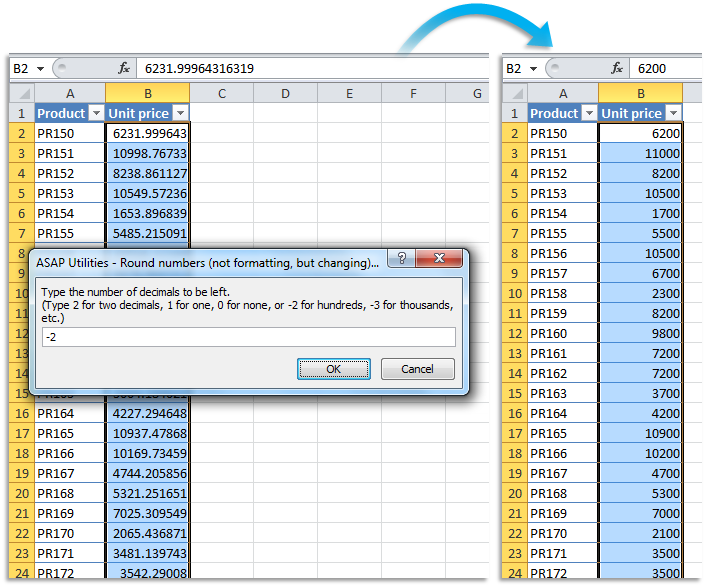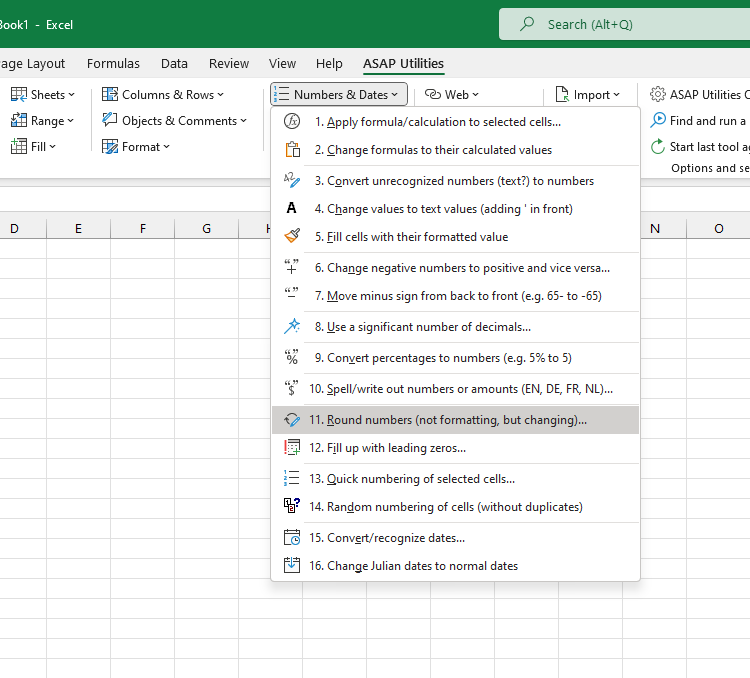# Round numbers (not formatting, but changing)...

English (us)NederlandsDeutschEspañolFrançaisPortuguês do BrasilItalianoРусский中文(简体)日本語

## Numbers & Dates  ›  11. Round numbers (not formatting, but changing)...

This utility replaces the numbers in the selected cells with the rounded values.
Instead of rounding the number by using cell formatting this tool will actually change the value in the cells.

The utility will ask you to give a number to specify how to round, similar to Excel's =ROUND() function:
• positive number: rounds a number to a specified number of digits.
• zero: rounds the value to the nearest integer.
• negative number: the number is rounded to the left of the decimal point for example to tens, hundreds, thousands etc.

For example a cell with the value 105.326 rounded to 1 will be changed to 105.3, rounded to 0 it will become 105 and rounded to -1 it will become 110.

## Screenshots

Example screenshot: A Round the selected numbers to 2 decimalsExample screenshot: B Round the selected numbers to the nearest integer (zero decimal places)Example screenshot: C Round the selected numbers to the nearest multiple of 100